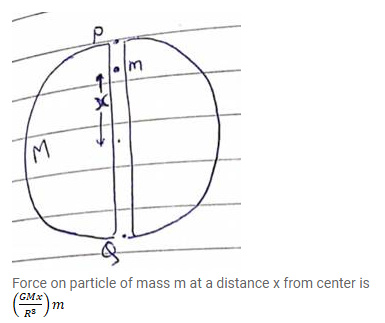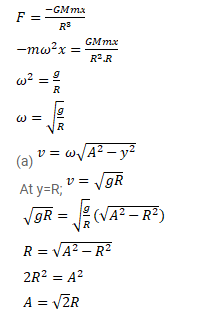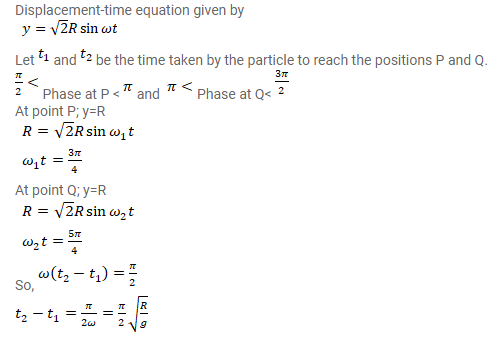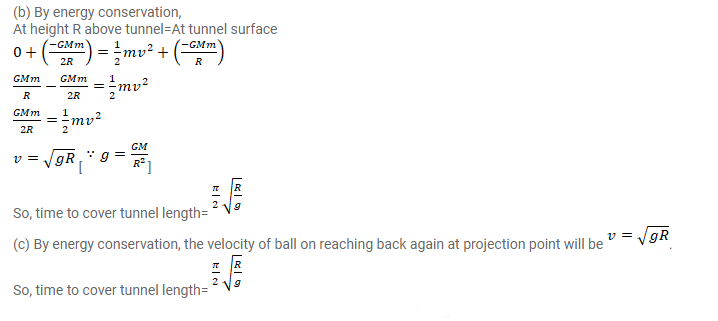# Assume that a tunnel is dug across the earth (radius=R) passing through its center.

Question:

Assume that a tunnel is dug across the earth (radius=R) passing through its center. Find the time a particle takes to cover the length of the tunnel if

(a) it is projected into the tunnel with a speed of $\sqrt{g R}$

(b) it is released from a height $\mathrm{R}$ above the tunnel

(c) it is thrown vertically upward along the length of tunnel with a speed of $\sqrt{g R}$.

Solution: